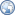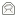## Introduction

This cipher was invented in the 16th century, and first written down by a French diplomat, Blaise de Vigenère. For a long time it was thought to be uncrackable, and it was popular for encoding sensitive data for transmission over the telegram system during the 19th century. It was first cracked by Kasiski in 1863.

I've written a program that allows you to encrypt and decrypt messages and files using the original form of the cipher, which only handles capital letters. It can also break the cipher - decode a ciphertext without the key - given enough text.

By today's standards the cipher is weak. Nonetheless, it is still used, slightly modified, in the "save document with password" function in some applications. The modification allows it to handle all bytes rather than just capital letters (by using XOR), but it still has the same vulnerabilities.

This program has been used to solve variousGeoCaches, for exampleCryptoCache Prime,Belaso's Stolen Cipher andYou Can't Get There From Here.

## Using the Program

The download package contains a Windows 32-bit executable, HTML documentation and C source code. So far, I've tested the code using DevStudio and gcc under Windows NT, but it should work on most platforms. If you are familiar with computers and basic cryptography then the program is largely self explanatory, although these points are worth highlighting:

• To work with a file, enter `!filename` when prompted for a string. The output is written to `filename.vig`
• At any prompts ending `(suggest ...)`, just press enter to use the suggested value
• While trying to work out an unknown key, a sample of the ciphertext is shown in lower case, alongside a sample of the uncovered plaintext in capitals.

## How the Cipher Works

The cipher needs a plaintext/ciphertext and a key to start. These are tidied by removing all non-alphabetic characters, and capitalising what remains. The tidied key must not be zero length. Firstly, each character in the text is assigned to a character in the key, like this:

PLAINTEXTMESSAGE
KEYKEYKEYKEYKEYK

Then, each character has a Caesar shift applied, with the amount of shift determined by the key character. The table below shows exactly what happens. To encrypt a character, find the column with the plaintext at the top, and the row with the key on the left side. The ciphertext is the character that this row and column intersect on. To decrypt, locate the row with the key on the left side, and look along this until you find the ciphertext. The plaintext is the character at the top of this column.

```  A B C D E F G H I J K L M N O P Q R S T U V W X Y Z
A A B C D E F G H I J K L M N O P Q R S T U V W X Y Z
B B C D E F G H I J K L M N O P Q R S T U V W X Y Z A
C C D E F G H I J K L M N O P Q R S T U V W X Y Z A B
D D E F G H I J K L M N O P Q R S T U V W X Y Z A B C
E E F G H I J K L M N O P Q R S T U V W X Y Z A B C D
F F G H I J K L M N O P Q R S T U V W X Y Z A B C D E
G G H I J K L M N O P Q R S T U V W X Y Z A B C D E F
H H I J K L M N O P Q R S T U V W X Y Z A B C D E F G
I I J K L M N O P Q R S T U V W X Y Z A B C D E F G H
J J K L M N O P Q R S T U V W X Y Z A B C D E F G H I
K K L M N O P Q R S T U V W X Y Z A B C D E F G H I J
L L M N O P Q R S T U V W X Y Z A B C D E F G H I J K
M M N O P Q R S T U V W X Y Z A B C D E F G H I J K L
N N O P Q R S T U V W X Y Z A B C D E F G H I J K L M
O O P Q R S T U V W X Y Z A B C D E F G H I J K L M N
P P Q R S T U V W X Y Z A B C D E F G H I J K L M N O
Q Q R S T U V W X Y Z A B C D E F G H I J K L M N O P
R R S T U V W X Y Z A B C D E F G H I J K L M N O P Q
S S T U V W X Y Z A B C D E F G H I J K L M N O P Q R
T T U V W X Y Z A B C D E F G H I J K L M N O P Q R S
U U V W X Y Z A B C D E F G H I J K L M N O P Q R S T
V V W X Y Z A B C D E F G H I J K L M N O P Q R S T U
W W X Y Z A B C D E F G H I J K L M N O P Q R S T U V
X X Y Z A B C D E F G H I J K L M N O P Q R S T U V W
Y Y Z A B C D E F G H I J K L M N O P Q R S T U V W X
Z Z A B C D E F G H I J K L M N O P Q R S T U V W X Y
```

The ciphertext for the above example works out to be:

ZPYSRROBRWIQCEEO

## Cracking the Cipher

Decrypting a ciphertext without a key is usually possible, provided that the message is substantially longer than the key (the program only works with keys of less than 255 characters), and the language of the plaintext is known (the program only works with English). Of course, the program can't be sure of what the key is - it can only find the most likely possibility. Because of this, information about each decision is presented to the user, who then has a chance to override the automatic suggestion.

The first step is to determine the length of the key, using the method of coincidence indexes. To calculate a coincidence index, shift the ciphertext and compare against itself, counting character matches:

CIPHERTEXT
CIPHERTEXT
----*-*

In this example, for a shift of three, there are two matches out of a possible seven, so the coincidence index is 28.57%. For random text the expected value is 3.85%, but this number tends to be higher for non-random text in any language, because some letters are more frequent than others. For Vigenere ciphertexts, the coincidence index is higher for shifts that are a multiple of the key length than otherwise (can you see why?). The program calculates the first 256 shifts, and finds the shortest length for which all shifts that are a multiple of this length have a coincidence index greater than 6%, which it suggests as the key length.

Knowing the key length, you can break the ciphertext up into chunks, each of which have been encrypted by the same character in the key. For each chunk, try decrypting it with each possible key letter (A...Z). Compare the letter frequencies in each possible plaintext with a table of English letter frequencies. The key letter which results in the least squared deviation is the most likely candidate, which the program suggests.

To test the cracking code, I used `/usr/share/dict/words` from a Linux system, a list of many English words. With this much ciphertext, keys as long as 200 characters can be uncovered without the user overriding any automatic suggestions.

## Program Design

• The general idea is to make the code look as if it were written in a functional language, like ML. This isn't rigorously enforced though, e.g. loops are used instead of deep recursion.
• Errors are handled by calling vig_error as soon as the error is detected. This uses setjmp/longjmp to escape back to the main menu, so there's no null return codes to worry about.
• vig_malloc is used instead of malloc. This keeps track of allocated memory, so calling vig_freeall frees everything.
• Functional style low-level routines are written, e.g. vig_loadfile takes a file name and loads it into newly allocated memory.
• For portability, size and endian of data types doesn't matter, and only POSIX system calls are used. The program assumes at least an 80 x 25 character terminal.
• Global variables are avoided, except where necessary in low level functions, e.g. storing the stack environment for error handling. Function names all have the prefix vig_.
© 1998 - 2012Paul Johnston, distributed under the BSD License   Updated:08 Jun 2009